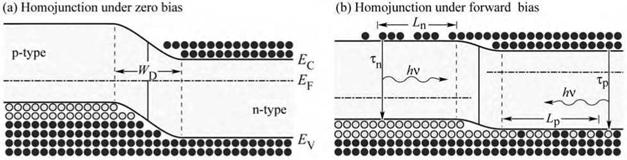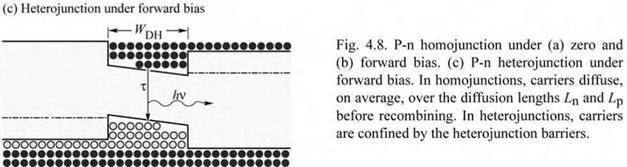## Carrier distribution in p-n homojunctions

The carrier distribution in p-n homojunctions, i. e. p-n junctions consisting of a single material, depends on the diffusion constant of the carriers. The diffusion constant of carriers is not easily measured. Much more common is the measurement of the carrier mobility; for example, by the Hall effect. The diffusion constant can be inferred from the carrier mobility by the Einstein relation, which, for non-degenerate semiconductors, is given by

kT kT

Dn = ------------------------- and-------- Dp = ----------- ^p. (4.18)

ee

Carriers injected into a neutral semiconductor, with no external electric field applied, propagate by diffusion. If carriers are injected into a region with opposite conductivity type, the minority carriers will eventually recombine. The mean distance a minority carrier diffuses before recombination is the diffusion length. Electrons injected into the p-type region will, on average, diffuse over the diffusion length Ln, before recombining with holes. The diffusion length is given by

Ln = VDn Tn and Lp = -jDp Tp (419)

where Tn and Tp are the electron and hole minority carrier lifetimes, respectively. In typical semiconductors, the diffusion length is of the order of a several micrometers. For example, the diffusion length of electrons in p-type GaAs is given by Ln = (220 cm2/s x 10-8 s)12 « 15 |im. Thus, minority carriers are distributed over a region several micrometers thick.

The distribution of carriers in a p-n junction under zero bias and under forward bias is shown in Figs. 4.8 (a) and (b), respectively. Note that minority carriers are distributed over quite a large distance. Furthermore, the minority carrier concentration decreases as these carriers diffuse further into the adjacent region. Thus recombination occurs over a large region, with a strongly changing minority carrier concentration. As will be shown below, the large recombination region in homojunctions is not beneficial for efficient recombination.Комментарии закрыты.